Asymptotic pseudounitary stacking operatorsNext: 1. Common-shot migration Up: EXAMPLES Previous: Datuming

## Migration

Least-squares migration, envisioned by Lailly (1984) and Tarantola (1984), has recently become a practical method and gained a lot of attention in the geophysical literature (Nemeth et al., 1999; Fomel et al., 2002; Chavent and Plessix, 1999; Duquet and Marfurt, 1999). Using the theory of asymptotic pseudo-unitary operators allows us to reconcile this approach with the method of asymptotic true-amplitude migration (Bleistein et al., 2001).

As recognized by Tygel et al. (1994), true-amplitude migration (Schleicher et al., 1993; Goldin, 1992) is the asymptotic inversion of seismic modeling represented by the Kirchhoff high-frequency approximation. The Kirchhoff approximation for a reflected wave (Bleistein, 1984; Haddon and Buchen, 1981) belongs to the class of stacking-type operators (1) with the summation path(41)

the weighting function(42)

and the additional time filter. Here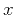denotes a point at the reflector surface,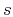is the source location, andis the receiver location at the observation surface. The parametercorresponds to the configuration of observation. That is,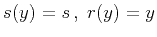for the common-shot configuration,for the zero-offset configuration, and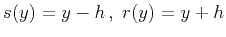for the common-offset configuration (whereis the half-offset). The functionsandhave the same meaning as in the datuming example, representing the one-way traveltime and the one-way geometric spreading, respectively. The functionis known as the obliquity factor. Its definition is(43)

where the anglesand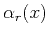are formed by the incident and reflected waves with the normal to the reflector at the point, andand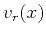are the corresponding velocities in the vicinity of this point. In this paper, I leave the case of converted (e.g., P-SV) waves outside the scope of consideration and assume thatequals(e.g., in P-P reflection). In this case, it is important to notice that at the stationary point of the Kirchhoff integral,(the law of reflection), and therefore(44)

The stationary point of the Kirchhoff integral is the point where the stacking curve (41) is tangent to the actual reflection traveltime curve. When our goal is asymptotic inversion, it is appropriate to use equation (44) in place of (43) to construct the inverse operator. The weighted function (42) can include other factors affecting the leading-order (WKBJ) ray amplitude, such as the source signature, caustics counter (the KMAH-index), and transmission coefficient for the interfaces (Chapman and Drummond, 1982; Cerveny, 2001). In the following analysis, I neglect these factors for simplicity.

The modelimplied by the Kirchhoff modeling integral is the wavefield with the wavelet shape of the incident wave and the amplitude proportional to the reflector coefficient along the reflector surface. The goal of true-amplitude migration is to recoverfrom the observed seismic data. In order to obtain the image of the reflectors, the reconstructed model is evaluated at the timeequal to zero. The Kirchhoff modeling integral requires explicit definition of the reflector surface. However, its inverse doesn't require explicit specification of the reflector location. For each point of the subsurface, one can find the normal to the hypothetical reflector by bisecting the angle between theandrays. Born scattering approximation provides a different physical model for the reflected waves. According to this approximation, the recorded waves are viewed as scattered on smooth local inhomogeneities rather than reflected from sharp reflector surfaces. The inversion of Born modeling (Miller et al., 1987; Bleistein, 1987) closely corresponds with the result of Kirchhoff integral inversion. For an unknown reflector and the correct macro-velocity model, the asymptotic inversion reconstructs the signal located at the reflector surface with the amplitude proportional to the reflector coefficient.

As follows from the form of the summation path (41), the integral migration operator must have the summation path(45)

to reconstruct the geometry of the reflector at the migrated section. According to equation (8), the asymptotic reconstruction of the wavelet requires, in addition, the derivative filter. The asymptotic reconstruction of the amplitude defines the true-amplitude weighting function in accordance with equation (10), as follows:(46)

The weighting function of the asymptotic pseudo-unitary migration is found analogously to equation (34) as(47)

Unlike true-amplitude migration, this type of migration operator does not change the dimensionality of the input. Several specific cases exist for different configurations of the input data.

SubsectionsAsymptotic pseudounitary stacking operatorsNext: 1. Common-shot migration Up: EXAMPLES Previous: Datuming

2013-03-03